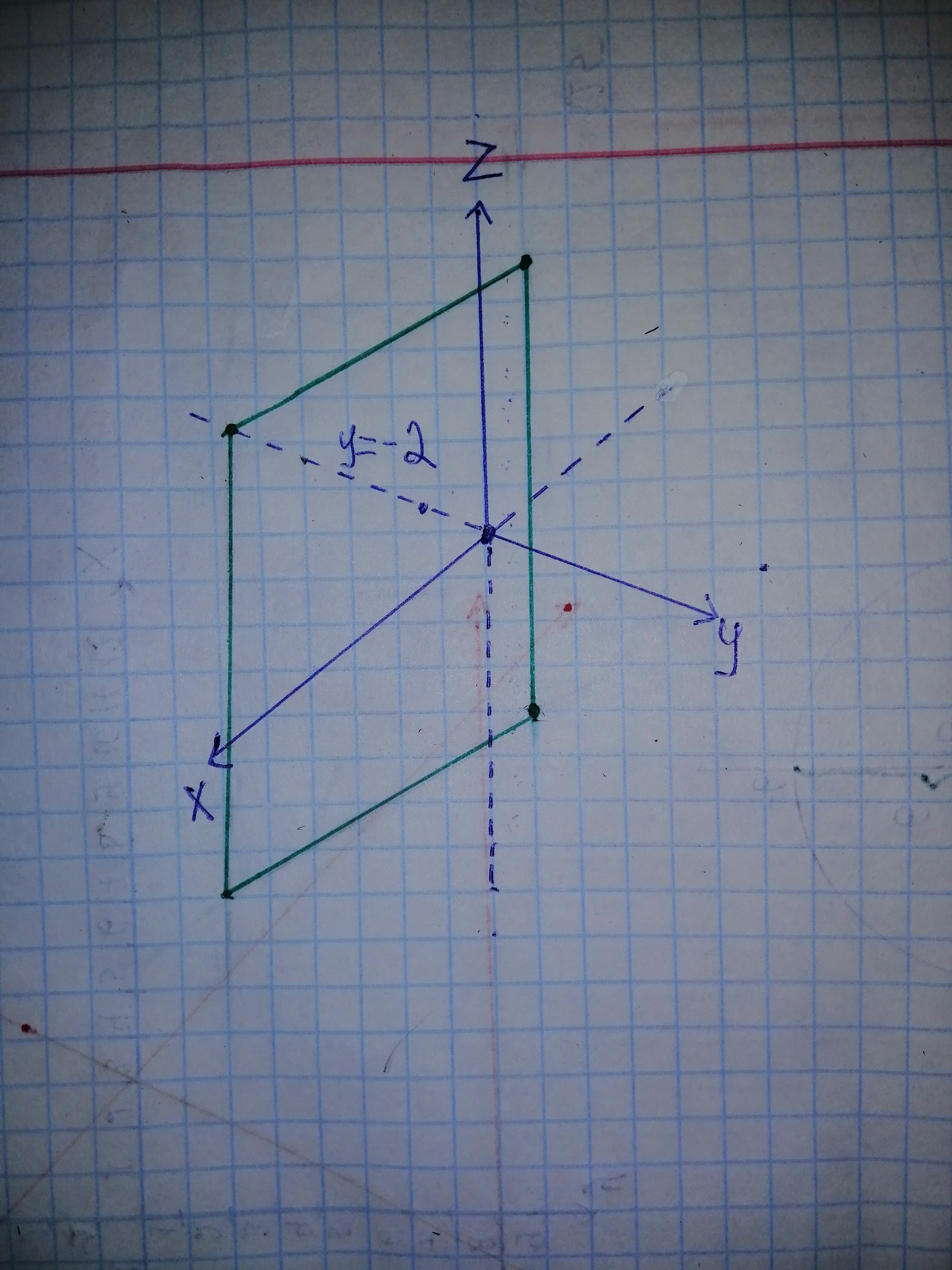Ask question

# Descibe in words the region of RR^{3} represented by the equation or inequality.y = -2# Descibe in words the region of RR^{3} represented by the equation or inequality.y = -2

Question
Alternate coordinate systemsasked 2021-03-02

Descibe in words the region of $$\mathbb{R^{3}}$$ represented by the equation or inequality.
$$y = -2$$

## Answers (1)2021-03-03

The equation $$y = -2\ in\ \mathbb{R^{3}}$$ represenrs the vertical plane that is parallel to the xz - plane and it locates 2 units left to the xz - plane.
$$RR^{3}$$ is the three dimensional coordinate system which contains x, y and z - coordinates.
The equation $$y = -2\ in\ \mathbb{R^{3}}$$
represents the set $${(x, y, z)|y = -2}$$,
which is the set of all points in $$\mathbb{R^{3}}$$ whose y - coordinate is -2 and x, z - coordinates are any values.
The equation $$y = -2\ in\ \mathbb{R^{3}}$$ is sketched as shown in Figure 1From Figure 1, the equation $$y = -2\ in\ \mathbb{R^{3}}$$ represents the vertical plane that is parallel to the xz - plane and it locates 2 units left to the xz - plane.
Thus, the equation $$y = -2\ in\ \mathbb{R^{3}}$$ is described.

### Relevant Questionsasked 2021-01-30

A line L through the origin in $$\displaystyle\mathbb{R}^{{3}}$$ can be represented by parametric equations of the form x = at, y = bt, and z = ct. Use these equations to show that L is a subspase of $$RR^3$$  by showing that if $$v_1=(x_1,y_1,z_1)\ and\ v_2=(x_2,y_2,z_2)$$  are points on L and k is any real number, then $$kv_1\ and\ v_1+v_2$$  are also points on L.asked 2021-01-31

(10%) In $$R^2$$, there are two sets of coordinate systems, represented by two distinct bases: $$(x_1, y_1)$$ and $$(x_2, y_2)$$. If the equations of the same ellipse represented by the two distinct bases are described as follows, respectively: $$2(x_1)^2 -4(x_1)(y_1) + 5(y_1)^2 - 36 = 0$$ and $$(x_2)^2 + 6(y_2)^2 - 36 = 0.$$ Find the transformation matrix between these two coordinate systems: $$(x_1, y_1)$$ and $$(x_2, y_2)$$.asked 2021-01-02

Solve the given Alternate Coordinate Systems and give a correct answer 10) Convert the equation from Cartesian to polar coordinates solving for $$r^2$$:
$$\frac{x^2}{9} - \frac{y^2}{16} = 25$$asked 2020-12-28

Assume that T is a linear transformation. Find the standard matrix of T.
$$\displaystyle{T}=\mathbb{R}^{{2}}\rightarrow\mathbb{R}^{{4}}\ {s}{u}{c}{h} \ {t}\hat \ {{T}}{\left({e}_{{1}}\right)}={\left({7},{1},{7},{1}\right)},{\quad\text{and}\quad}{T}{\left({e}_{{2}}\right)}={\left(-{8},{5},{0},{0}\right)},{w}{h}{e}{r}{e}{\ e}_{{1}}={\left({1},{0}\right)},{\quad\text{and}\quad}{e}_{{2}}={\left({0},{1}\right)}$$.asked 2021-02-08

To find: The equivalent polar equation for the given rectangular-coordinate equation.
Given:
$$\displaystyle{x}^{2}+{y}^{2}+{8}{x}={0}$$asked 2020-11-05

To graph: The sketch of the solution set of system of nonlinear inequality
$$\displaystyle{\left[{x}^{{{2}}}\ +\ {y}^{{{2}}}\geq\ {9}\right.}]$$
$$\displaystyle{x}^{{{2}}}\ +\ {y}^{{{2}}}\leq\ {25}$$
$$\displaystyle{y}\geq\ {\left|{x}\right|}$$asked 2020-11-12

The given system of inequality:
$$\displaystyle{\left\lbrace\begin{matrix}{y}<{9}-{x}^{2}\\{y}\ge{x}+{3}\end{matrix}\right.}$$
Also find the coordinates of all vertices, and check whether the solution set is bounded.asked 2021-01-31

The quadratic function $$\displaystyle{y}={a}{x}^{2}+{b}{x}+{c}$$ whose graph passes through the points (1, 4), (2, 1) and (3, 4).asked 2020-10-21

To solve:
$$\displaystyle{\left(\begin{matrix}{x}-{2}{y}={2}\\{2}{x}+{3}{y}={11}\\{y}-{4}{z}=-{7}\end{matrix}\right)}$$asked 2021-02-26

The reason ehy the point $$(-1, \frac{3\pi}{2})$$ lies on the polar graph $$r=1+\cos \theta$$ even though it does not satisfy the equation.

...